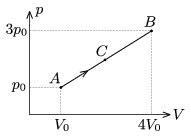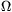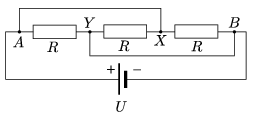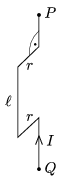Mathematical and Physical Journal
for High Schools
Issued by the MATFUND Foundation
 Already signed up? New to KöMaL?

# KöMaL Problems in Physics, January 2010

Show/hide problems of signs:## Problems with sign 'M'

Deadline expired on February 10, 2010.

M. 302. Measure the coefficients of static and dynamic friction between a sleigh and the snow. (If there is no snow, between the sleigh and the ground.)

(6 pont)

statistics## Problems with sign 'P'

Deadline expired on February 10, 2010.

P. 4214. A freight train, undergoing uniform motion, passes the semaphore next to the rails in 4 seconds. Then it passes a 40-m long train, which moves with the same speed as the freight train but into the opposite direction, also in 4 seconds. What is the speed of the freight train in km/h?

(3 pont)

solution (in Hungarian), statistics

P. 4215. A body is attached to a vertically hung soft spring. The body is released and the time during which the body reaches the lowest point of its path is measured. How many times does this time more than the time while an object, which falls freely without any initial speed, covers the same distance?

(4 pont)

solution (in Hungarian), statistics

P. 4216. A sample of Helium gas is taken through the process AB, shown in the figure, p0=105 Pa, V0=3 dm3. Determine the pressure and the volume of the gas at state C, until which QAC=3700 J heat is added to the gas.(5 pont)

solution (in Hungarian), statistics

P. 4217. If the pair of plates, which is to deflect the charges, in the cathode ray tube is uncharged, then the electrons, which are ejected from the electron gun, travel through the tube with a constant velocity and they hit the screen with this speed. What can be stated about the speed of the electrons with which they hit the screen when there is a constant amount of charge on the pair of plates?

(5 pont)

solution (in Hungarian), statistics

P. 4218. What is the current in the wires which join the points A and X, and the points X and Y, shown in the figure? Data: R=6, U=24 V.(4 pont)

solution (in Hungarian), statistics

P. 4219. The piece of rigid wire, shown in the figure, and which can freely turn about the vertical axis of PQ, is placed into uniform horizontal magnetic field. B=0.05 T, r=0.05 m,=0.3 m. A current of I=10 A flows in the wire and the mass of one metre-long wire is 10 dkg. What is the period of this piece of wire when it is oscillating with small amplitude?(5 pont)

solution (in Hungarian), statistics

P. 4220. An immersion heater is rated at 350 W when it is run on a sinusoidal electricity supply of voltage 230 V.

a) What is the smallest and the greatest instantaneous power of the immersion heater when it is operating?

b) What is the maximum value of the instantaneous current that flows through the heater?

c) Why is it not allowed to turn on the heater before immersing it into the water?

(3 pont)

solution (in Hungarian), statistics

P. 4221. The base of a glass prism is an isosceles triangle. The angle between the equal sides is 40o and the refractive index of the glass of the prism is 1.5. What should the angle of incidence of the light beam incident on one of the congruent faces of the prism be in order that the refracted ray is to be parallel to the base of the isosceles triangle. What is the answer if the prism is placed into water of refractive index of 1.33, or into bensole of refractive index 1.6?

(4 pont)

solution (in Hungarian), statistics

P. 4222. Three times ionized UF6 molecules are accelerated through a voltage of 5 kV, and then through a small hole they are led to a region of magnetic field of induction 1 T. The induction vector is perpendicular to the velocity of the molecules.

a) What is the path of the ions? Give the characteristic parameters of the paths of the ions which contain the 238 and the 235 isotopes of the Uranium.

b) In this way the two Uranium isotopes can be separated. During the Second World War the available ion sources could produce an ion current of 1 mA. How long would it have taken to separate 1 kg pure 235U isotope with this method?

(4 pont)

solution (in Hungarian), statistics

P. 4223. A high energy proton collides with a 73Li nucleus of negligible speed. The angle between the emitted 2 alpha particles is 90o. At least what was the speed of the proton?

(5 pont)

solution (in Hungarian), statistics